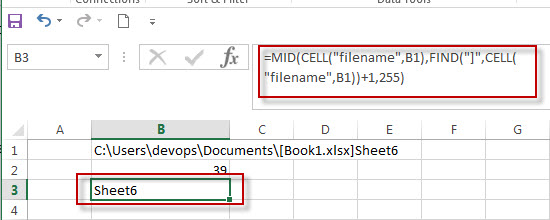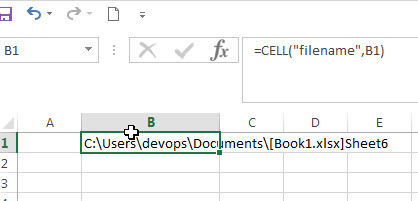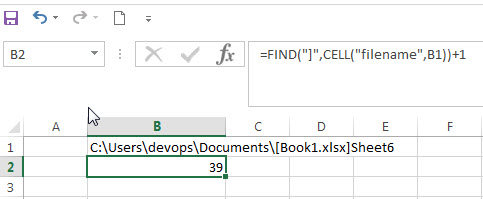# Get the Current Worksheet Name only

If you want to get the current worksheet name only in excel, you can use a combination of the MID function, the CELL function and FIND function. You can use the below generic formula:

`=MID(CELL("filename",B1),FIND("]",CELL("filename",B1))+1,255)`The below explains how the above excel formula works:

The CELL function will get the full information of the current worksheet contain the full file name and its path.

=CELL(“filename”,B1)The FIND function will locate the position of the right bracket symbol in the result returned by CELL function.

So the starting position of the filename of the current workbook can be got from the below formula:

`=FIND("]",CELL("filename",B1))+1`The MID function will extract the worksheet name from a text string returned by CELL function at the position that returned by the FIND function.

### Related Functions

• Excel Find function
The Excel FIND function returns the position of the first text string (sub string) from the first character of the second text string.The FIND function is a build-in function in Microsoft Excel and it is categorized as a Text Function.The syntax of the FIND function is as below:= FIND  (find_text, within_text,[start_num])…
• Excel MID function
The Excel MID function returns a substring from a text string at the position that you specify.The syntax of the MID function is as below:= MID (text, start_num, num_chars)…
• Excel CELL function
The Excel CELL function returns information about the formatting, location, size, or contents of a cell.The syntax of the CELL function is as below:= CELL (info_type,[reference])…
Related Posts

How to Convert Text to Time in Excel

This post will guide you how to convert time string to time in Excel. Assuming that you have a list of text string in your worksheet, and you wish to convert these time string to standard time format, how to ...

How to Extract Initials From a Name in Excel

This post will guide you how to get initials from a given name using a formula in Excel. How do I extract initials from names in Excel 2013/2016. Extract Initials from a Name Using a Formula Extract Initials from a ...

How to Extract Number from Text String in Excel

This post will guide you how to extract number from a given test string in Excel. How do I extract all numbers from string using a formula in Excel. How to get all number from a given test string using ...

How to Convert mmddyy to Date in Excel

This post will guide you how to convert non-standard date formats or text to a standard date in Excel. If you have a date with mmddyy format, and you want to change it as the normal date format as mm/dd/yyyy ...

How to Convert Military Time to Standard Time in Excel

This post will guide you how to convert military time to standard time with a formula in Excel. How do I convert from military time 17:29:30 to regular time 5:29:30PM in Excel.  How do I Convert Standard time to military ...

How to Extract Text between Two Text Strings in Excel

This post will guide you how to extract text between two given text strings in Excel. How do I get text string between two words with a formula in Excel. Extract Text between Two Text Strings Assuming that you have ...

How to Remove Numbers from Text in Excel

This post will guide you how to remove all numbers from a text string in one cell in Excel. How do I strip all numeric characters from a given cell with a formula in Excel. How do I remove numbers ...

How to Split Word into Different Cells in Excel

This post will guide you how to split word into different columns with a formula in Excel. How do I split word or number into separate cells with VBA Macro code in Excel 2010/1013/2016. Split Word into Different Cells with ...

Insert Character or Text to Cells

This post will guide you how to insert character or text in middle of cells in Excel. How do I add text string or character to each cell of a column or range with a formula in Excel. How to ...

Reverse Concatenate Formula in Excel

This post will guide youw how to reverse the concatenate function in Excel. how do I split the text string into the muliptle cells with a formula in Excel. How to split text data of a cell to multiple cells ...

Sidebar